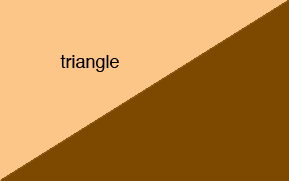SEARCH HOMEMath Central Quandaries & QueriesQuestion from s, a student: how to construct a triangle whose area is equal to the area of a given square?Hi,

If you start with a rectangle and cut it along a diagonal you get a triangle with half the area of the rectangle.Thus you can start with a square, expand it to a rectangle with twice the area and then cut it along a diagonal to obtain a triangle with the same area as the original square.

I hope this helps,
PennyMath Central is supported by the University of Regina and The Pacific Institute for the Mathematical Sciences.